# Tag: Riemann

Don’t be fooled by introductory remarks to the effect that ‘the field with one element was conceived by Jacques Tits half a century ago, etc. etc.’

While this is a historic fact, and, Jacques Tits cannot be given enough credit for bringing a touch of surrealism into mathematics, but this is not the main drive for people getting into F_un, today.

There is a much deeper and older motivation behind most papers published recently on $\mathbb{F}_1$. Few of the authors will be willing to let you in on the secret, though, because if they did, it would sound much too presumptuous…

So, let’s have it out into the open : F_un mathematics’ goal is no less than proving the Riemann Hypothesis.

And even then, authors hide behind a smoke screen. The ‘official’ explanation being “we would like to copy Weil’s proof of the Riemann hypothesis in the case of function fields of curves over finite fields, by considering spec(Z) as a ‘curve’ over an algebra ‘dessous’ Z namely $\mathbb{F}_1$”. Alas, at this moment, none of the geometric approaches over the field with one element can make this stick.

Believe me for once, the main Jugendtraum of most authors is to get a grip on cyclotomy over $\mathbb{F}_1$. It is no accident that Connes makes a dramatic pauze in his YouTubeVideo to let the viewer see this equation on the backboard

$\mathbb{F}_{1^n} \otimes_{\mathbb{F}_1} \mathbb{Z} = \mathbb{Z}[x]/(x^n-1)$

But, what is the basis of all this childlike enthusiasm? A somewhat concealed clue is given in the introduction of the Kapranov-Smirnov paper. They write :

“In [?] the affine line over $\mathbb{F}_1$ was considered; it consists formally of 0 and all the roots of unity. Put slightly differently, this leads to the consideration of “algebraic extensions” of $\mathbb{F}_1$. By analogy with genuine finite fields we would like to think that there is exactly one such extension of any given degree n, denote it by $\mathbb{F}_{1^n}$.

Of course, $\mathbb{F}_{1^n}$ does not exist in a rigorous sense, but we can think if a scheme $X$ contains n-th roots of unity, then it is defined over $\mathbb{F}_{1^n}$, so that there is a morphism

$p_X~:~X \rightarrow spec(\mathbb{F}_{1^n}$

The point of view that adjoining roots of unity is analogous to the extension of the base field goes back, at least to Weil (Lettre a Artin, Ouvres, vol 1) and Iwasawa…

Okay, so rush down to your library, pick out the first of three volumes of Andre Weil’s collected works, look up his letter to Emil Artin written on July 10th 1942 (19 printed pages!), and head for the final section. Weil writes :“Our proof of the Riemann hypothesis (in the function field case, red.) depended upon the extension of the function-fields by roots of unity, i.e. by constants; the way in which the Galois group of such extensions operates on the classes of divisors in the original field and its extensions gives a linear operator, the characteristic roots (i.e. the eigenvalues) of which are the roots of the zeta-function.

On a number field, the nearest we can get to this is by adjunction of $l^n$-th roots of unity, $l$ being fixed; the Galois group of this infinite extension is cyclic, and defines a linear operator on the projective limit of the (absolute) class groups of those successive finite extensions; this should have something to do with the roots of the zeta-function of the field. However, our extensions are ramified (but only at a finite number of places, viz. the prime divisors of $l$). Thus a preliminary study of similar problems in function-fields might enable one to guess what will happen in number-fields.”

A few years later, in 1947, he makes this a bit more explicit in his marvelous essay “L’avenir des mathematiques” (The future of mathematics). Weil is still in shell-shock after the events of the second WW, and writes in beautiful archaic French sentences lasting forever :

“L’hypothèse de Riemann, après qu’on eu perdu l’espoir de la démontrer par les méthodes de la théorie des fonctions, nous apparaît aujourd’hui sous un jour nouveau, qui la montre inséparable de la conjecture d’Artin sur les fonctions L, ces deux problèmes étant deux aspects d’une même question arithmético-algébrique, où l’étude simultanée de toutes les extensions cyclotomiques d’un corps de nombres donné jouera sans doute le rôle décisif.

L’arithmétique gausienne gravitait autour de la loi de réciprocité quadratique; nous savons maintenant que celle-ci n’est qu’un premier example, ou pour mieux dire le paradigme, des lois dites “du corps de classe”, qui gouvernent les extensions abéliennes des corps de nobres algébriques; nous savons formuler ces lois de manière à leur donner l’aspect d’un ensemble cohérent; mais, si plaisante à l’œil que soit cette façade, nous ne savons si elle ne masque pas des symmétries plus cachées.

Les automorphismes induits sur les groupes de classes par les automorphismes du corps, les propriétés des restes de normes dans les cas non cycliques, le passage à la limite (inductive ou projective) quand on remplace le corps de base par des extensions, par example cyclotomiques, de degré indéfiniment croissant, sont autant de questions sur lesquelles notre ignorance est à peu près complète, et dont l’étude contient peut-être la clef de l’hypothese de Riemann; étroitement liée à celles-ci est l’étude du conducteur d’Artin, et en particulier, dans le cas local, la recherche de la représentation dont la trace s’exprime au moyen des caractères simples avec des coefficients égaux aux exposants de leurs conducteurs.

Ce sont là quelques-unes des directions qu’on peut et qu’on doit songer à suivre afin de pénétrer dans le mystère des extensions non abéliennes; il n’est pas impossible que nous touchions là à des principes d’une fécondité extraordinaire, et que le premier pas décisif une fois fait dans cette voie doive nous ouvrir l’accès à de vastes domaines dont nous soupçonnons à peine l’existence; car jusqu’ici, pour amples que soient nos généralisations des résultats de Gauss, on ne peut dire que nus les ayons vraiment dépassés.”

It is perhaps surprising that Alain Connes and Katia Consani, two icons of noncommutative geometry, restrict themselves to define commutative algebraic geometry over $\mathbb{F}_1$, the field with one element.

My guess of why they stop there is as good as anyone’s. Perhaps they felt that there is already enough noncommutativity in Soule’s gadget-approach (the algebra $\mathcal{A}_X$ as in this post may very well be noncommutative). Perhaps they were only interested in the Bost-Connes system which can be entirely encoded in their commutative $\mathbb{F}_1$-geometry. Perhaps they felt unsure as to what the noncommutative scheme of an affine noncommutative algebra might be. Perhaps …

Remains the fact that their approach screams for a noncommutative extension. Their basic object is a covariant functor

$N~:~\mathbf{abelian} \rightarrow \mathbf{sets} \qquad A \mapsto N(A)$

from finite abelian groups to sets, together with additional data to the effect that there is a unique minimal integral scheme associated to $N$. In a series of posts on the Connes-Consani paper (starting here) I took some care of getting rid of all scheme-lingo and rephrasing everything entirely into algebras. But then, this set-up can be extended verbatim to noncommuative $\mathbb{F}_1$-geometry, which should start from a covariant functor

$N~:~\mathbf{groups} \rightarrow \mathbf{sets}$

from all finite groups to sets. Let’s recall quickly what the additional info should be making this functor a noncommutative (affine) F_un scheme :

There should be a finitely generated $\mathbb{C}$-algebra $R$ together with a natural transformation (the ‘evaluation’)

$e~:~N \rightarrow \mathbf{maxi}(R) \qquad N(G) \mapsto Hom_{\mathbb{C}-alg}(R, \mathbb{C} G)$

(both $R$ and the group-algebra $\mathbb{C} G$ may be noncommutative). The pair $(N, \mathbf{maxi}(R))$ is then called a gadget and there is an obvious notion of ‘morphism’ between gadgets.

The crucial extra ingredient is an affine $\mathbb{Z}$-algebra (possibly noncommutative) $S$
such that $N$ is a subfunctor of $\mathbf{mini}(S)~:~G \mapsto Hom_{\mathbb{Z}-alg}(S,\mathbb{Z} G)$ together with the following universal property :

any affine $\mathbb{Z}$-algebra $T$ having a gadget-morphism $~(N,\mathbf{maxi}(R)) \rightarrow (\mathbf{mini}(T),\mathbf{maxi}(T \otimes_{\mathbb{Z}} \mathbb{C}))$ comes from a $\mathbb{Z}$-algebra morphism $T \rightarrow S$. (If this sounds too cryptic for you, please read the series on C-C mentioned before).

So, there is no problem in defining noncommutative affine F_un-schemes. However, as with any generalization, this only makes sense provided (a) we get something new and (b) we have interesting examples, not covered by the restricted theory.

At first sight we do not get something new as in the only example we did in the C-C-series (the forgetful functor) it is easy to prove (using the same proof as given in this post) that the forgetful-functor $\mathbf{groups} \rightarrow \mathbf{sets}$ still has as its integral form the integral torus $\mathbb{Z}[x,x^{-1}]$. However, both theories quickly diverge beyond this example.

For example, consider the functor

$\mathbf{groups} \rightarrow \mathbf{sets} \qquad G \mapsto G \times G$

Then, if we restrict to abelian finite groups $\mathbf{abelian}$ it is easy to see (again by a similar argument) that the two-dimensional integer torus $\mathbb{Z}[x,y,x^{-1},y^{-1}]$ is the correct integral form. However, this algebra cannot be the correct form for the functor on the category of all finite groups as any $\mathbb{Z}$-algebra map $\phi~:~\mathbb{Z}[x,y,x^{-1},y^{-1}] \rightarrow \mathbb{Z} G$ determines (and is determined by) a pair of commuting units in $\mathbb{Z} G$, so the above functor can not be a subfunctor if we allow non-Abelian groups.

But then, perhaps there isn’t a minimal integral $\mathbb{Z}$-form for this functor? Well, yes there is. Take the free group in two letters (that is, all words in noncommuting $x,y,x^{-1}$ and $y^{-1}$ satisfying only the trivial cancellation laws between a letter and its inverse), then the corresponding integral group-algebra $\mathbb{Z} \mathcal{F}_2$ does the trick.

Again, the proof-strategy is the same. Given a gadget-morphism we have an algebra map $f~:~T \mapsto \mathbb{C} \mathcal{F}_2$ and we have to show, using the universal property that the image of $T$ is contained in the integral group-algebra $\mathbb{Z} \mathcal{F}_2$. Take a generator
$z$ of $T$ then the degree of the image $f(z)$ is bounded say by $d$ and we can always find a subgroup $H \subset \mathcal{F}_2$ such that $\mathcal{F}_2/H$ is a fnite group and the quotient map $\mathbb{C} \mathcal{F}_2 \rightarrow \mathbb{C} \mathcal{F}_2/H$ is injective on the subspace spanned by all words of degree strictly less than $d+1$. Then, the usual diagram-chase finishes the proof.

What makes this work is that the free group $\mathcal{F}_2$ has ‘enough’ subgroups of finite index, a property it shares with many interesting discrete groups. Whence the blurb-message :

if the integers $\mathbb{Z}$ see a discrete group $\Gamma$, then the field $\mathbb{F}_1$ sees its profinite completion $\hat{\Gamma} = \underset{\leftarrow}{lim}~\Gamma/ H$So, yes, we get something new by extending the Connes-Consani approach to the noncommutative world, but do we have interesting examples? As “interesting” is a subjective qualification, we’d better invoke the authority-argument.

Alexander Grothendieck (sitting on the right, manifestly not disputing a vacant chair with Jean-Pierre Serre, drinking on the left (a marvelous picture taken by F. Hirzebruch in 1958)) was pushing the idea that profinite completions of arithmetical groups were useful in the study of the absolute Galois group $Gal(\overline{\mathbb{Q}}/\mathbb{Q})$, via his theory of dessins d’enfants (children;s drawings).

In a previous life, I’ve written a series of posts on dessins d’enfants, so I’ll restrict here to the basics. A smooth projective $\overline{\mathbb{Q}}$-curve $X$ has a Belyi-map $X \rightarrow \mathbb{P}^1_{\overline{\mathbb{Q}}}$ ramified only in three points ${ 0,1,\infty }$. The “drawing” corresponding to $X$ is a bipartite graph, drawn on the Riemann surface $X_{\mathbb{C}}$ obtained by lifting the unit interval $[0,1]$ to $X$. As the absolute Galois group acts on all such curves (and hence on their corresponding drawings), the action of it on these dessins d’enfants may give us a way into the multiple mysteries of the absolute Galois group.

In his “Esquisse d’un programme” (Sketch of a program if you prefer to read it in English) he writes :

“C’est ainsi que mon attention s’est portée vers ce que j’ai appelé depuis la “géométrie algêbrique anabélienne”, dont le point de départ est justement une étude (pour le moment limitée à la caractéristique zéro) de l’action de groupe de Galois “absolus” (notamment les groupes $Gal(\overline{K}/K)$, ou $K$ est une extension de type fini du corps premier) sur des groupes fondamentaux géométriques (profinis) de variétés algébriques (définies sur $K$), et plus particulièrement (rompant avec une tradition bien enracinée) des groupes fondamentaux qui sont trés éloignés des groupes abéliens (et que pour cette raison je nomme “anabéliens”). Parmi ces groupes, et trés proche du groupe $\hat{\pi}_{0,3}$, il y a le compactifié profini du groupe modulaire $SL_2(\mathbb{Z})$, dont le quotient par le centre $\pm 1$ contient le précédent comme sous-groupe de congruence mod 2, et peut s’interpréter d’ailleurs comme groupe “cartographique” orienté, savoir celui qui classifie les cartes orientées triangulées (i.e. celles dont les faces des triangles ou des monogones).”

and a bit further, he writes :

“L’élément de structure de $SL_2(\mathbb{Z})$ qui me fascine avant tout, est bien sur l’action extérieure du groupe de Galois $Gal(\overline{\mathbb{Q}}/\mathbb{Q})$ sur le compactifié profini. Par le théorème de Bielyi, prenant les compactifiés profinis de sous-groupes d’indice fini de $SL_2(\mathbb{Z})$, et l’action extérieure induite (quitte à passer également à un sous-groupe overt de $Gal(\overline{\mathbb{Q}},\mathbb{Q})$), on trouve essentiellement les groupes fondamentaux de toutes les courbes algébriques définis sur des corps de nombres $K$, et l’action extérieure de $Gal(\overline{K}/K)$ dessus.”

So, is there a noncommutative affine variety over $\mathbb{F}_1$ of which the unique minimal integral model is the integral group algebra of the modular group $\mathbb{Z} \Gamma$ (with $\Gamma = PSL_2(\mathbb{Z})$? Yes, here it is

$N_{\Gamma}~:~\mathbf{groups} \rightarrow \mathbf{sets} \qquad G \mapsto G_2 \times G_3$

where $G_n$ is the set of all elements of order $n$ in $G$. The reason behind this is that the modular group is the free group product $C_2 \ast C_3$.

Fine, you may say, but all this is just algebra. Where is the noncommutative complex variety or the noncommutative integral scheme in all this? Well, we can introduce them too but as this post is already 1300 words long, I’ll better leave this for another time. In case you cannot stop thinking about it, here’s the short answer.

The complex noncommutative variety has as its ‘points’ all finite dimensional simple complex representations of the modular group, and the ‘points’ of the noncommutative $\mathbb{F}_1$-scheme are exactly the (modular) dessins d’enfants…

The Monster is the largest of the 26 sporadic simple groups and has order

808 017 424 794 512 875 886 459 904 961 710 757 005 754 368 000 000 000

= 2^46 3^20 5^9 7^6 11^2 13^3 17 19 23 29 31 41 47 59 71.

It is not so much the size of its order that makes it hard to do actual calculations in the monster, but rather the dimensions of its smallest non-trivial irreducible representations (196 883 for the smallest, 21 296 876 for the next one, and so on).

In characteristic two there is an irreducible representation of one dimension less (196 882) which appears to be of great use to obtain information. For example, Robert Wilson used it to prove that The Monster is a Hurwitz group. This means that the Monster is generated by two elements g and h satisfying the relations

$g^2 = h^3 = (gh)^7 = 1$

Geometrically, this implies that the Monster is the automorphism group of a Riemann surface of genus g satisfying the Hurwitz bound 84(g-1)=#Monster. That is,

g=9619255057077534236743570297163223297687552000000001=42151199 * 293998543 * 776222682603828537142813968452830193

Or, in analogy with the Klein quartic which can be constructed from 24 heptagons in the tiling of the hyperbolic plane, there is a finite region of the hyperbolic plane, tiled with heptagons, from which we can construct this monster curve by gluing the boundary is a specific way so that we get a Riemann surface with exactly 9619255057077534236743570297163223297687552000000001 holes. This finite part of the hyperbolic tiling (consisting of #Monster/7 heptagons) we’ll call the empire of the monster and we’d love to describe it in more detail.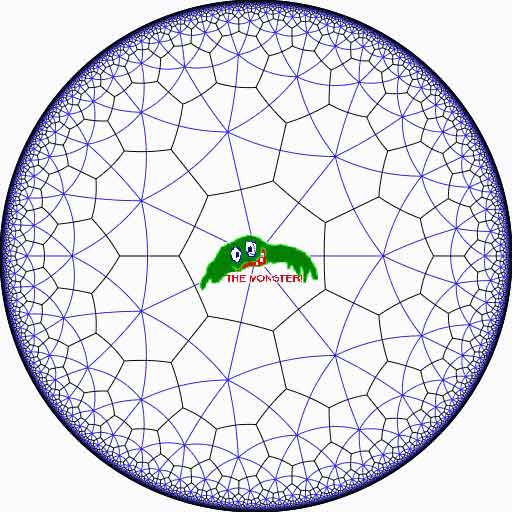Look at the half-edges of all the heptagons in the empire (the picture above learns that every edge in cut in two by a blue geodesic). They are exactly #Monster such half-edges and they form a dessin d’enfant for the monster-curve.

If we label these half-edges by the elements of the Monster, then multiplication by g in the monster interchanges the two half-edges making up a heptagonal edge in the empire and multiplication by h in the monster takes a half-edge to the one encountered first by going counter-clockwise in the vertex of the heptagonal tiling. Because g and h generated the Monster, the dessin of the empire is just a concrete realization of the monster.

Because g is of order two and h is of order three, the two permutations they determine on the dessin, gives a group epimorphism $C_2 \ast C_3 = PSL_2(\mathbb{Z}) \rightarrow \mathbb{M}$ from the modular group $PSL_2(\mathbb{Z})$ onto the Monster-group.

In noncommutative geometry, the group-algebra of the modular group $\mathbb{C} PSL_2$ can be interpreted as the coordinate ring of a noncommutative manifold (because it is formally smooth in the sense of Kontsevich-Rosenberg or Cuntz-Quillen) and the group-algebra of the Monster $\mathbb{C} \mathbb{M}$ itself corresponds in this picture to a finite collection of ‘points’ on the manifold. Using this geometric viewpoint we can now ask the question What does the Monster see of the modular group?

To make sense of this question, let us first consider the commutative equivalent : what does a point P see of a commutative variety X?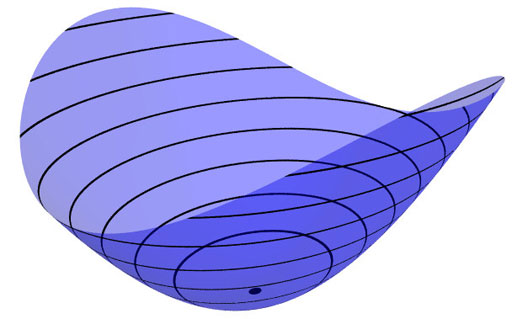Evaluation of polynomial functions in P gives us an algebra epimorphism $\mathbb{C}[X] \rightarrow \mathbb{C}$ from the coordinate ring of the variety $\mathbb{C}[X]$ onto $\mathbb{C}$ and the kernel of this map is the maximal ideal $\mathfrak{m}_P$ of
$\mathbb{C}[X]$ consisting of all functions vanishing in P.

Equivalently, we can view the point $P= \mathbf{spec}~\mathbb{C}[X]/\mathfrak{m}_P$ as the scheme corresponding to the quotient $\mathbb{C}[X]/\mathfrak{m}_P$. Call this the 0-th formal neighborhood of the point P.

This sounds pretty useless, but let us now consider higher-order formal neighborhoods. Call the affine scheme $\mathbf{spec}~\mathbb{C}[X]/\mathfrak{m}_P^{n+1}$ the n-th forml neighborhood of P, then the first neighborhood, that is with coordinate ring $\mathbb{C}[X]/\mathfrak{m}_P^2$ gives us tangent-information. Alternatively, it gives the best linear approximation of functions near P.
The second neighborhood $\mathbb{C}[X]/\mathfrak{m}_P^3$ gives us the best quadratic approximation of function near P, etc. etc.

These successive quotients by powers of the maximal ideal $\mathfrak{m}_P$ form a system of algebra epimorphisms

$\ldots \frac{\mathbb{C}[X]}{\mathfrak{m}_P^{n+1}} \rightarrow \frac{\mathbb{C}[X]}{\mathfrak{m}_P^{n}} \rightarrow \ldots \ldots \rightarrow \frac{\mathbb{C}[X]}{\mathfrak{m}_P^{2}} \rightarrow \frac{\mathbb{C}[X]}{\mathfrak{m}_P} = \mathbb{C}$

and its inverse limit $\underset{\leftarrow}{lim}~\frac{\mathbb{C}[X]}{\mathfrak{m}_P^{n}} = \hat{\mathcal{O}}_{X,P}$ is the completion of the local ring in P and contains all the infinitesimal information (to any order) of the variety X in a neighborhood of P. That is, this completion $\hat{\mathcal{O}}_{X,P}$ contains all information that P can see of the variety X.

In case P is a smooth point of X, then X is a manifold in a neighborhood of P and then this completion
$\hat{\mathcal{O}}_{X,P}$ is isomorphic to the algebra of formal power series $\mathbb{C}[[ x_1,x_2,\ldots,x_d ]]$ where the $x_i$ form a local system of coordinates for the manifold X near P.

Right, after this lengthy recollection, back to our question what does the monster see of the modular group? Well, we have an algebra epimorphism

$\pi~:~\mathbb{C} PSL_2(\mathbb{Z}) \rightarrow \mathbb{C} \mathbb{M}$

and in analogy with the commutative case, all information the Monster can gain from the modular group is contained in the $\mathfrak{m}$-adic completion

$\widehat{\mathbb{C} PSL_2(\mathbb{Z})}_{\mathfrak{m}} = \underset{\leftarrow}{lim}~\frac{\mathbb{C} PSL_2(\mathbb{Z})}{\mathfrak{m}^n}$

where $\mathfrak{m}$ is the kernel of the epimorphism $\pi$ sending the two free generators of the modular group $PSL_2(\mathbb{Z}) = C_2 \ast C_3$ to the permutations g and h determined by the dessin of the pentagonal tiling of the Monster’s empire.

As it is a hopeless task to determine the Monster-empire explicitly, it seems even more hopeless to determine the kernel $\mathfrak{m}$ let alone the completed algebra… But, (surprise) we can compute $\widehat{\mathbb{C} PSL_2(\mathbb{Z})}_{\mathfrak{m}}$ as explicitly as in the commutative case we have $\hat{\mathcal{O}}_{X,P} \simeq \mathbb{C}[[ x_1,x_2,\ldots,x_d ]]$ for a point P on a manifold X.

Here the details : the quotient $\mathfrak{m}/\mathfrak{m}^2$ has a natural structure of $\mathbb{C} \mathbb{M}$-bimodule. The group-algebra of the monster is a semi-simple algebra, that is, a direct sum of full matrix-algebras of sizes corresponding to the dimensions of the irreducible monster-representations. That is,

$\mathbb{C} \mathbb{M} \simeq \mathbb{C} \oplus M_{196883}(\mathbb{C}) \oplus M_{21296876}(\mathbb{C}) \oplus \ldots \ldots \oplus M_{258823477531055064045234375}(\mathbb{C})$

with exactly 194 components (the number of irreducible Monster-representations). For any $\mathbb{C} \mathbb{M}$-bimodule $M$ one can form the tensor-algebra

$T_{\mathbb{C} \mathbb{M}}(M) = \mathbb{C} \mathbb{M} \oplus M \oplus (M \otimes_{\mathbb{C} \mathbb{M}} M) \oplus (M \otimes_{\mathbb{C} \mathbb{M}} M \otimes_{\mathbb{C} \mathbb{M}} M) \oplus \ldots \ldots$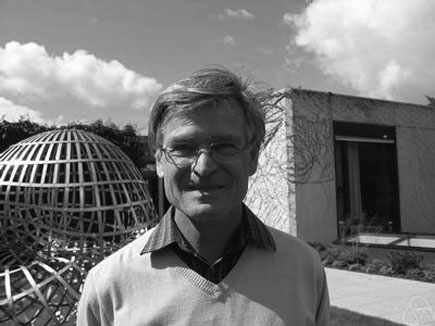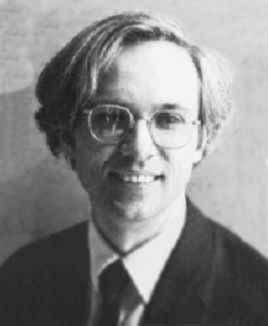and applying the formal neighborhood theorem for formally smooth algebras (such as $\mathbb{C} PSL_2(\mathbb{Z})$) due to Joachim Cuntz (left) and Daniel Quillen (right) we have an isomorphism of algebras

$\widehat{\mathbb{C} PSL_2(\mathbb{Z})}_{\mathfrak{m}} \simeq \widehat{T_{\mathbb{C} \mathbb{M}}(\mathfrak{m}/\mathfrak{m}^2)}$

where the right-hand side is the completion of the tensor-algebra (at the unique graded maximal ideal) of the $\mathbb{C} \mathbb{M}$-bimodule $\mathfrak{m}/\mathfrak{m}^2$, so we’d better describe this bimodule explicitly.

Okay, so what’s a bimodule over a semisimple algebra of the form $S=M_{n_1}(\mathbb{C}) \oplus \ldots \oplus M_{n_k}(\mathbb{C})$? Well, a simple S-bimodule must be either (1) a factor $M_{n_i}(\mathbb{C})$ with all other factors acting trivially or (2) the full space of rectangular matrices $M_{n_i \times n_j}(\mathbb{C})$ with the factor $M_{n_i}(\mathbb{C})$ acting on the left, $M_{n_j}(\mathbb{C})$ acting on the right and all other factors acting trivially.

That is, any S-bimodule can be represented by a quiver (that is a directed graph) on k vertices (the number of matrix components) with a loop in vertex i corresponding to each simple factor of type (1) and a directed arrow from i to j corresponding to every simple factor of type (2).

That is, for the Monster, the bimodule $\mathfrak{m}/\mathfrak{m}^2$ is represented by a quiver on 194 vertices and now we only have to determine how many loops and arrows there are at or between vertices.

Using Morita equivalences and standard representation theory of quivers it isn’t exactly rocket science to determine that the number of arrows between the vertices corresponding to the irreducible Monster-representations $S_i$ and $S_j$ is equal to

$dim_{\mathbb{C}}~Ext^1_{\mathbb{C} PSL_2(\mathbb{Z})}(S_i,S_j)-\delta_{ij}$

Now, I’ve been wasting a lot of time already here explaining what representations of the modular group have to do with quivers (see for example here or some other posts in the same series) and for quiver-representations we all know how to compute Ext-dimensions in terms of the Euler-form applied to the dimension vectors.

Right, so for every Monster-irreducible $S_i$ we have to determine the corresponding dimension-vector $~(a_1,a_2;b_1,b_2,b_3)$ for the quiver

$\xymatrix{ & & & & \vtx{b_1} \\ \vtx{a_1} \ar[rrrru]^(.3){B_{11}} \ar[rrrrd]^(.3){B_{21}} \ar[rrrrddd]_(.2){B_{31}} & & & & \\ & & & & \vtx{b_2} \\ \vtx{a_2} \ar[rrrruuu]_(.7){B_{12}} \ar[rrrru]_(.7){B_{22}} \ar[rrrrd]_(.7){B_{23}} & & & & \\ & & & & \vtx{b_3}}$

Now the dimensions $a_i$ are the dimensions of the +/-1 eigenspaces for the order 2 element g in the representation and the $b_i$ are the dimensions of the eigenspaces for the order 3 element h. So, we have to determine to which conjugacy classes g and h belong, and from Wilson’s paper mentioned above these are classes 2B and 3B in standard Atlas notation.

So, for each of the 194 irreducible Monster-representations we look up the character values at 2B and 3B (see below for the first batch of those) and these together with the dimensions determine the dimension vector $~(a_1,a_2;b_1,b_2,b_3)$.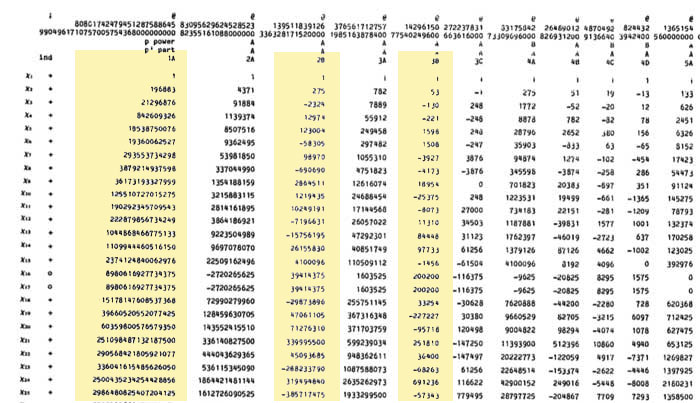For example take the 196883-dimensional irreducible. Its 2B-character is 275 and the 3B-character is 53. So we are looking for a dimension vector such that $a_1+a_2=196883, a_1-275=a_2$ and $b_1+b_2+b_3=196883, b_1-53=b_2=b_3$ giving us for that representation the dimension vector of the quiver above $~(98579,98304,65663,65610,65610)$.

Okay, so for each of the 194 irreducibles $S_i$ we have determined a dimension vector $~(a_1(i),a_2(i);b_1(i),b_2(i),b_3(i))$, then standard quiver-representation theory asserts that the number of loops in the vertex corresponding to $S_i$ is equal to

$dim(S_i)^2 + 1 – a_1(i)^2-a_2(i)^2-b_1(i)^2-b_2(i)^2-b_3(i)^2$

and that the number of arrows from vertex $S_i$ to vertex $S_j$ is equal to

$dim(S_i)dim(S_j) – a_1(i)a_1(j)-a_2(i)a_2(j)-b_1(i)b_1(j)-b_2(i)b_2(j)-b_3(i)b_3(j)$

This data then determines completely the $\mathbb{C} \mathbb{M}$-bimodule $\mathfrak{m}/\mathfrak{m}^2$ and hence the structure of the completion $\widehat{\mathbb{C} PSL_2}_{\mathfrak{m}}$ containing all information the Monster can gain from the modular group.

But then, one doesn’t have to go for the full regular representation of the Monster. Any faithful permutation representation will do, so we might as well go for the one of minimal dimension.

That one is known to correspond to the largest maximal subgroup of the Monster which is known to be a two-fold extension $2.\mathbb{B}$ of the Baby-Monster. The corresponding permutation representation is of dimension 97239461142009186000 and decomposes into Monster-irreducibles

$S_1 \oplus S_2 \oplus S_4 \oplus S_5 \oplus S_9 \oplus S_{14} \oplus S_{21} \oplus S_{34} \oplus S_{35}$

(in standard Atlas-ordering) and hence repeating the arguments above we get a quiver on just 9 vertices! The actual numbers of loops and arrows (I forgot to mention this, but the quivers obtained are actually symmetric) obtained were found after laborious computations mentioned in this post and the details I’ll make avalable here.

Anyone who can spot a relation between the numbers obtained and any other part of mathematics will obtain quantities of genuine (ie. non-Inbev) Belgian beer…

Next week, our annual summer school Geometric and Algebraic Methods with Applications in Physics will start, once again (ive lost count which edition it is).

Because Isar is awol to la douce France, I’ll be responsible (once again) for the web-related stuff of the meeting. So, here a couple of requests to participants/lecturers :

• if you are giving a mini-course and would like to have your material online, please contact me and i’ll make you an author of the Arts blog.
• if you are a student attending the summerschool and would love to do some Liveblogging about the meeting, please do the same.

I’ll try to do some cross-posting here when it comes to my own lectures (and, perhaps, a few others). For now, I settled on ‘What is noncommutative geometry?’ as a preliminary title, but then, I’m in the position to change the program with a few keystrokes, so I’ll probably change it by then (or remove myself from it altogether…).

At times, I feel it would be more fun to do a few talks on Math-blogging. An entertaining hour could be spend on the forensic investigation of the recent Riemann-Hypothesis-hype in (a good part of) the math-blogosphere

We are after the geometric trinity corresponding to the trinity of exceptional Galois groupsThe surfaces on the right have the corresponding group on the left as their group of automorphisms. But, there is a lot more group-theoretic info hidden in the geometry. Before we sketch the $L_2(11)$ case, let us recall the simpler situation of $L_2(7)$.

There are some excellent web-page on the Klein quartic and it would be too hard to try to improve on them, so we refer to John Baez’ page and Greg Egan’s page for more details.

The Klein quartic is the degree 4 projective plane curve defined by the equation $x^3y+y^3z+z^3x=0$. It can be tiled with a set of 24 regular heptagons, or alternatively with a set of 56 equilateral triangles and these two tilings are dual to each other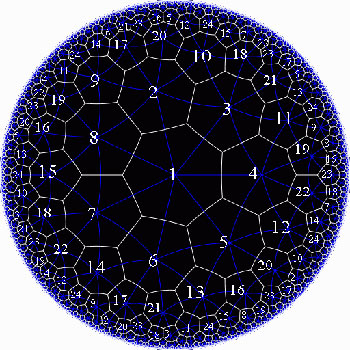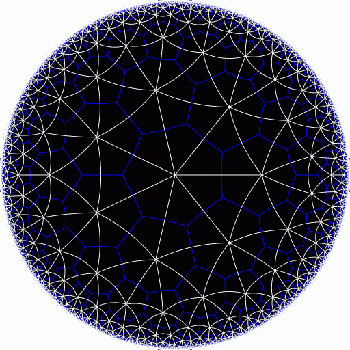In the triangular tiling, there are 56 triangles, 84 edges and 24 vertices. The 56 triangles come in 7 bunches of 8 each and we give the 7 bunches of triangles each a different color as in the pictures below made by Greg Egan. Observe that in the hyperbolic tiling all triangles look alike, but in the picture on the left most of them get warped as we try to embed the quartic in 3-space (which is impossible to do properly). The non-warped triangles (the red ones) come into pairs, the top and bottom triangles of a triangular prism, one prism at each of the four ‘vertices’ of a tetrahedron.

The automorphism group $L_2(7)$ acts on these triangles as $S_4$ acts on the triangles in a truncated cube.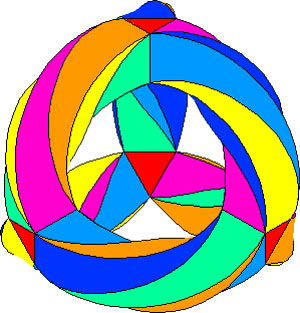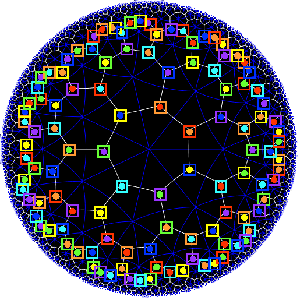The buckyball construction from a conjugacy class of order 11 elements from $L_2(11)$ recalled last time, has an analogon $L_2(7)$, leading to the truncated cube.

In $L_2(7)$ there are two conjugacy classes of subgroups isomorphic to $S_4$ (the rotation-symmetry group of the cube) as well as two conjugacy classes of order 7 elements, each consisting of precisely 24 elements, say C and D. The normalizer subgroup of C has order 21, so there is a cyclic group of order 3 acting non-trivially on the conjugacy class C with 8 orbits consisting of three elements each. These are the eight triangles of the truncated cube identified above as the red triangles.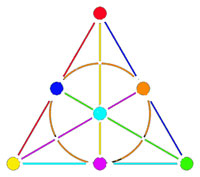Shifting perspective, we can repeat this for each of the seven different colors. That is, we have seven truncated cubes in the Klein quartic. On each of them a copy of $S_4$ acts and these subgroups form one of the two conjugacy classes of $S_4$ in the group $L_2(7)$. The colors of the triangles of these seven truncated cubes are indicated by bullets in the picture above on the right. The other conjugacy class of $S_4$’s act on ‘truncated anti-cubes’ which also come in seven bunches of which the color is indicated by a square in that picture.

If you spend enough time on it you will see that each (truncated) cube is completely disjoint from precisely 3 (truncated) anti-cubes. This reminds us of the Fano-plane (picture on the left) : it has 7 points (our seven truncated cubes), 7 lines (the truncated anti-cubes) and the incidence relation of points and lines corresponds to the disjointness of (truncated) cubes and anti-cubes! This is the geometric interpretation of the group-theoretic realization that $L_2(7) \simeq PGL_3(\mathbb{F}_2)$ is the isomorphism group of the projective plane over the finite field $\mathbb{F}_2$ on two elements, that is, the Fano plane. The colors of the picture on the left indicate the colors of cubes (points) and anti-cubes (lines) consistent with Egan’s picture above.

Further, the 24 vertices correspond to the 24 cusps of the modular group $\Gamma(7)$. Recall that a modular interpretation of the Klein quartic is as $\mathbb{H}/\Gamma(7)$ where $\mathbb{H}$ is the upper half-plane on which the modular group $\Gamma = PSL_2(\mathbb{Z})$ acts via Moebius transformations, that is, to a 2×2 matrix corresponds the transformation

$$\begin{bmatrix} a & b \\ c & d \end{bmatrix}$$ <----> $z \mapsto \frac{az+b}{cz+d}$

Okay, now let’s briefly sketch the exciting results found by Pablo Martin and David Singerman in the paper From biplanes to the Klein quartic and the buckyball, extending the above to the group $L_2(11)$.

There is one important modification to be made. Recall that the Cayley-graph to get the truncated cube comes from taking as generators of the group $S_4$ the set ${ (3,4),(1,2,3) }$, that is, an order two and an order three element, defining an epimorphism from the modular group $\Gamma= C_2 \ast C_3 \rightarrow S_4$.

We have also seen that in order to get the buckyball as a Cayley-graph for $A_5$ we need to take the generating set ${ (2,3)(4,5),(1,2,3,4,5) }$, so a degree two and a degree five element.

Hence, if we want to have a corresponding Riemann surface we’d better not start from the action of the modular group on the upper half-plane, but rather the action via Moebius transformations of the
Hecke group

$H^5 \simeq C_2 \ast C_5 = \langle z \mapsto -\frac{1}{z}, z \mapsto z+ \phi \rangle$

where $\phi = \frac{1 + \sqrt{5}}{2}$ is the golden ratio.

But then, there is an epimorphism $H^5 \rightarrow L_2(11)$ (as this group is generated by one element of degree 2 and one of degree 5) and let $\Lambda$ denote its kernel. Observe that $\Lambda$ is the analogon of the modular subgroup $\Gamma(7)$ used above to define the Klein quartic.

Hence, Martin and Singerman define the buckyball curve as the modular quotient $X=\mathbb{H}/\Lambda$ which is a Riemann surface of genus 70.

The terminlogy is motivated by the fact that, precisely as we got 7 truncated cubes in the Klein quartic, we now get 11 truncated icosahedra (that is, buckyballs) in $X$. The 11 coming, analogous to the Klein case, from thefact that there are precisely two conjugacy classes of subgroups of $L_2(11)$ isomorphic to $A_5$, each class containing precisely eleven elements!
The 60 vertices of the buckyball again correspond to the fact that there are 60 cusps in this case.

So, what is the analogon of the Fano plane in this case? Well, observe that the Fano-plane is a biplane of order two. That is, if we take as ‘points’ the points of the Fano plane and as ‘lines’ the complements of lines in the Fano plane then this defines a biplane structure. This means that any two distinct ‘points’ are contained in two distinct ‘lines’ and that two distinct ‘lines’ intersect in two distinct ‘points’. A biplane is said to be of order k is each ‘line’ consist of k-2 ‘points’. As the complement of a line in the Fano plane consists of 4 points, the Fano plane is therefore a biplane of order 2. The intersection pattern of cubes and anti-cubes in the Klein quartic is this biplane structure on the Fano plane.

In a similar way, Martin and Singerman show that the two conjugacy classes of subgroups isomorphic to $A_5$ in $L_2(11)$, each containing exactly 11 elements, correspond to 11 embedded buckyballs (and 11 anti-buckyballs) in the buckyball-curve $X$ and that the intersection relations among them describe the combinatorial structure of a biplane of order three if we view the 11 buckys as ‘points’ and the anti-buckys as ‘lines’.

That is, the buckyball curve is a perfect geometric counterpart of the Klein quartic for the two trinitiesAt the Arcadian Functor, Kea also has a post on this in which she conjectures that the Kac-Moody algebra of E11 may be related to the buckyball curve.

References :

David Singerman, “Klein’s Riemann surface of genus 3 and regular embeddings of finite projective planes” Bull. London Math. Soc. 18 (1986) 364-370.

Pablo Martin and David Singerman, “From biplanes to the Klein quartic and the Buckyball” (note that this is a preliminary version, please contact David Singerman for the latest version).

We saw that the icosahedron can be constructed from the alternating group $A_5$ by considering the elements of a conjugacy class of order 5 elements as the vertices and edges between two vertices if their product is still in the conjugacy class.

This description is so nice that one would like to have a similar construction for the buckyball. But, the buckyball has 60 vertices, so they surely cannot correspond to the elements of a conjugacy class of $A_5$. But, perhaps there is a larger group, somewhat naturally containing $A_5$, having a conjugacy class of 60 elements?

This is precisely the statement contained in Galois’ last letter. He showed that 11 is the largest prime p such that the group $L_2(p)=PSL_2(\mathbb{F}_p)$ has a (transitive) permutation presentation on p elements. For, p=11 the group $L_2(11)$ is of order 660, so it permuting 11 elements means that this set must be of the form $X=L_2(11)/A$ with $A \subset L_2(11)$ a subgroup of 60 elements… and it turns out that $A \simeq A_5$…

Actually there are TWO conjugacy classes of subgroups isomorphic to $A_5$ in $L_2(11)$ and we have already seen one description of these using the biplane geometry (one class is the stabilizer subgroup of a ‘line’, the other the stabilizer subgroup of a point).

Here, we will give yet another description of these two classes of $A_5$ in $L_2(11)$, showing among other things that the theory of dessins d’enfant predates Grothendieck by 100 years.

In the very same paper containing the first depiction of the Dedekind tessellation, Klein found that there should be a degree 11 cover $\mathbb{P}^1_{\mathbb{C}} \rightarrow \mathbb{P}^1_{\mathbb{C}}$ with monodromy group $L_2(11)$, ramified only in the three points ${ 0,1,\infty }$ such that there is just one point lying over $\infty$, seven over 1 of which four points where two sheets come together and finally 5 points lying over 0 of which three where three sheets come together. In 1879 he wanted to determine this cover explicitly in the paper “Ueber die Transformationen elfter Ordnung der elliptischen Funktionen” (Math. Annalen) by describing all Riemann surfaces with this ramification data and pick out those with the correct monodromy group.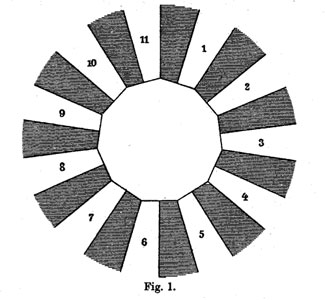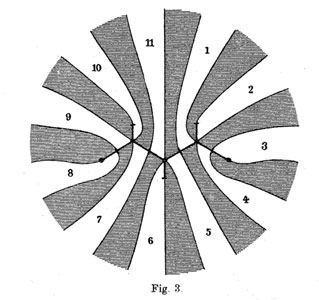He manages to do so by associating to all these covers their ‘dessins d’enfants’ (which he calls Linienzuges), that is the pre-image of the interval [0,1] in which he marks the preimages of 0 by a bullet and those of 1 by a +, such as in the innermost darker graph on the right above. He even has these two wonderful pictures explaining how the dessin determines how the 11 sheets fit together. (More examples of dessins and the correspondences of sheets were drawn in the 1878 paper.)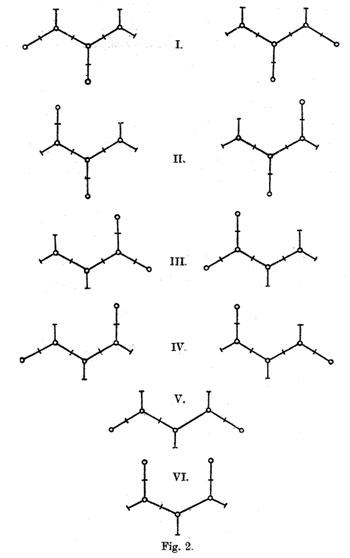The ramification data translates to the following statements about the Linienzuge : (a) it must be a tree ($\infty$ has one preimage), (b) there are exactly 11 (half)edges (the degree of the cover),
(c) there are 7 +-vertices and 5 o-vertices (preimages of 0 and 1) and (d) there are 3 trivalent o-vertices and 4 bivalent +-vertices (the sheet-information).

Klein finds that there are exactly 10 such dessins and lists them in his Fig. 2 (left). Then, he claims that one the two dessins of type I give the correct monodromy group. Recall that the monodromy group is found by giving each of the half-edges a number from 1 to 11 and looking at the permutation $\tau$ of order two pairing the half-edges adjacent to a +-vertex and the order three permutation $\sigma$ listing the half-edges by cycling counter-clockwise around a o-vertex. The monodromy group is the group generated by these two elements.

Fpr example, if we label the type V-dessin by the numbers of the white regions bordering the half-edges (as in the picture Fig. 3 on the right above) we get
$\sigma = (7,10,9)(5,11,6)(1,4,2)$ and $\tau=(8,9)(7,11)(1,5)(3,4)$.

Nowadays, it is a matter of a few seconds to determine the monodromy group using GAP and we verify that this group is $A_{11}$.

Of course, Klein didn’t have GAP at his disposal, so he had to rule out all these cases by hand.

gap> g:=Group((7,10,9)(5,11,6)(1,4,2),(8,9)(7,11)(1,5)(3,4));
Group([ (1,4,2)(5,11,6)(7,10,9), (1,5)(3,4)(7,11)(8,9) ])
gap> Size(g);
19958400
gap> IsSimpleGroup(g);
true

Klein used the fact that $L_2(11)$ only has elements of orders 1,2,3,5,6 and 11. So, in each of the remaining cases he had to find an element of a different order. For example, in type V he verified that the element $\tau.(\sigma.\tau)^3$ is equal to the permutation (1,8)(2,10,11,9,6,4,5)(3,7) and consequently is of order 14.

Perhaps Klein knew this but GAP tells us that the monodromy group of all the remaining 8 cases is isomorphic to the alternating group $A_{11}$ and in the two type I cases is indeed $L_2(11)$. Anyway, the two dessins of type I correspond to the two conjugacy classes of subgroups $A_5$ in the group $L_2(11)$.

But, back to the buckyball! The upshot of all this is that we have the group $L_2(11)$ containing two classes of subgroups isomorphic to $A_5$ and the larger group $L_2(11)$ does indeed have two conjugacy classes of order 11 elements containing exactly 60 elements (compare this to the two conjugacy classes of order 5 elements in $A_5$ in the icosahedral construction). Can we construct the buckyball out of such a conjugacy class?

To start, we can identify the 12 pentagons of the buckyball from a conjugacy class C of order 11 elements. If $x \in C$, then so do $x^3,x^4,x^5$ and $x^9$, whereas the powers ${ x^2,x^6,x^7,x^8,x^{10} }$ belong to the other conjugacy class. Hence, we can divide our 60 elements in 12 subsets of 5 elements and taking an element x in each of these, the vertices of a pentagon correspond (in order) to $~(x,x^3,x^9,x^5,x^4)$.

Group-theoretically this follows from the fact that the factorgroup of the normalizer of x modulo the centralizer of x is cyclic of order 5 and this group acts naturally on the conjugacy class of x with orbits of size 5.

Finding out how these pentagons fit together using hexagons is a lot subtler… and in The graph of the truncated icosahedron and the last letter of Galois Bertram Kostant shows how to do this.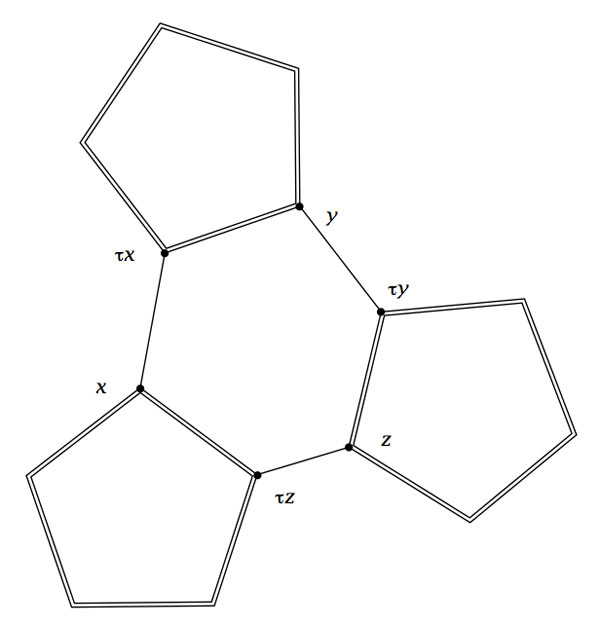Fix a subgroup isomorphic to $A_5$ and let D be the set of all its order 2 elements (recall that they form a full conjugacy class in this $A_5$ and that there are precisely 15 of them). Now, the startling observation made by Kostant is that for our order 11 element $x$ in C there is a unique element $a \in D$ such that the commutator$~b=[x,a]=x^{-1}a^{-1}xa$ belongs again to D. The unique hexagonal side having vertex x connects it to the element $b.x$which belongs again to C as $b.x=(ax)^{-1}.x.(ax)$.

Concluding, if C is a conjugacy class of order 11 elements in $L_2(11)$, then its 60 elements can be viewed as corresponding to the vertices of the buckyball. Any element $x \in C$ is connected by two pentagonal sides to the elements $x^{3}$ and $x^4$ and one hexagonal side connecting it to $\tau x = b.x$.

The buckyball is without doubt the hottest mahematical object at the moment (at least in Europe). Recall that the buckyball (middle) is a mixed form of two Platonic solids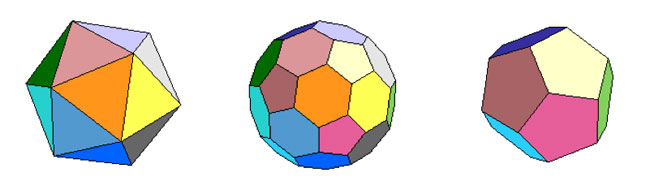the Icosahedron on the left and the Dodecahedron on the right.

For those of you who don’t know anything about football, it is that other ball-game, best described via a quote from the English player Gary Lineker

“Football is a game for 22 people that run around, play the ball, and one referee who makes a slew of mistakes, and in the end Germany always wins.”

We still have a few days left hoping for a better ending… Let’s do some bucky-maths : what is the rotation symmetry group of the buckyball?

For starters, dodeca- and icosahedron are dual solids, meaning that if you take the center of every face of a dodecahedron and connect these points by edges when the corresponding faces share an edge, you’ll end up with the icosahedron (and conversely). Therefore, both solids (as well as their mixture, the buckyball) will have the same group of rotational symmetries. Can we at least determine the number of these symmetries?

Take the dodecahedron and fix a face. It is easy to find a rotation taking this face to anyone of its five adjacent faces. In group-slang : the rotation automorphism group acts transitively on the 12 faces of the dodecohedron. Now, how many of them fix a given face? These can only be rotations with axis through the center of the face and there are exactly 5 of them preserving the pentagonal face. So, in all we have $12 \times 5 = 60$ rotations preserving any of the three solids above. By composing two of its elements, we get another rotational symmetry, so they form a group and we would like to determine what that group is.

There is one group that springs to mind $A_5$, the subgroup of all even permutations on 5 elements. In general, the alternating group has half as many elements as the full permutation group $S_n$, that is $\frac{1}{2} n!$ (for multiplying with the involution (1,2) gives a bijection between even and odd permutations). So, for $A_5$ we get 60 elements and we can list them :

• the trivial permutation$~()$, being the identity.
• permutations of order two with cycle-decompostion $~(i_1,i_2)(i_3,i_4)$, and there are exactly 15 of them around when all numbers are between 1 and 5.
• permutations of order three with cycle-form $~(i_1,i_2,i_3)$ of which there are exactly 20.
• permutations of order 5 which have to form one full cycle $~(i_1,i_2,i_3,i_4,i_5)$. There are 24 of those.

Can we at least view these sets of elements as rotations of the buckyball? Well, a dodecahedron has 12 pentagobal faces. So there are 4 nontrivial rotations of order 5 for every 2 opposite faces and hence the dodecaheder (and therefore also the buckyball) has indeed 6×4=24 order 5 rotational symmetries.

The icosahedron has twenty triangles as faces, so any of the 10 pairs of opposite faces is responsible for two non-trivial rotations of order three, giving us 10×2=20 order 3 rotational symmetries of the buckyball.

The order two elements are slightly harder to see. The icosahedron has 30 edges and there is a plane going through each of the 15 pairs of opposite edges splitting the icosahedron in two. Hence rotating to interchange these two edges gives one rotational symmetry of order 2 for each of the 15 pairs.

And as 24+20+15+1(identity) = 60 we have found all the rotational symmetries and we see that they pair up nicely with the elements of $A_5$. But do they form isomorphic groups? In other words, can the buckyball see the 5 in the group $A_5$.

In a previous post I’ve shown that one way to see this 5 is as the number of inscribed cubes in the dodecahedron. But, there is another way to see the five based on the order 2 elements described before.

If you look at pairs of opposite edges of the icosahedron you will find that they really come in triples such that the planes determined by each pair are mutually orthogonal (it is best to feel this on ac actual icosahedron). Hence there are 15/3 = 5 such triples of mutually orthogonal symmetry planes of the icosahedron and of course any rotation permutes these triples. It takes a bit of more work to really check that this action is indeed the natural permutation action of $A_5$ on 5 elements.

Having convinced ourselves that the group of rotations of the buckyball is indeed the alternating group $A_5$, we can reverse the problem : can the alternating group $A_5$ see the buckyball???

Well, for starters, it can ‘see’ the icosahedron in a truly amazing way. Look at the conjugacy classes of $A_5$. We all know that in the full symmetric group $S_n$ elements belong to the same conjugacy class if and only if they have the same cycle decomposition and this is proved using the fact that the conjugation f a cycle $~(i_1,i_2,\ldots,i_k)$ under a permutation $\sigma \in S_n$ is equal to the cycle $~(\sigma(i_1),\sigma(i_2),\ldots,\sigma(i_k))$ (and this gives us also the candidate needed to conjugate two partitions into each other).

Using this trick it is easy to see that all the 15 order 2 elements of $A_5$ form one conjugacy class, as do the 20 order 3 elements. However, the 24 order 5 elements split up in two conjugacy classes of 12 elements as the permutation needed to conjugate $~(1,2,3,4,5)$ to $~(1,2,3,5,4)$ is $~(4,5)$ but this is not an element of $A_5$.

Okay, now take one of these two conjugacy classes of order 5 elements, say that of $~(1,2,3,4,5)$. It consists of 12 elements, 12 being also the number of vertices of the icosahedron. So, is there a way to identify the elements in the conjugacy class to the vertices in such a way that we can describe the edges also in terms of group-computations in $A_5$?

Surprisingly, this is indeed the case as is demonstrated in a marvelous paper by Kostant “The graph of the truncated icosahedron and the last letter of Galois”.

Two elements $a,b$ in the conjugacy class C share an edge if and only if their product $a.b \in A_5$ still belongs to the conjugacy class C!

So, for example $~(1,2,3,4,5).(2,1,4,3,5) = (2,5,4)$ so there is no edge between these elements, but on the other hand $~(1,2,3,4,5).(5,3,4,1,2)=(1,5,2,4,3)$ so there is an edge between these! It is no coincidence that $~(5,3,4,1,2)=(2,1,4,3,5)^{-1}$ as inverse elements correspond in the bijection to opposite vertices and for any pair of non-opposite vertices of an icosahedron it is true that either they are neighbors or any one of them is the neighbor of the opposite vertex of the other element.

If we take $u=(1,2,3,4,5)$ and $v=(5,3,4,1,2)$ (or any two elements of the conjugacy class such that u.v is again in the conjugacy class), then one can describe all the vertices of the icosahedron group-theoretically as follows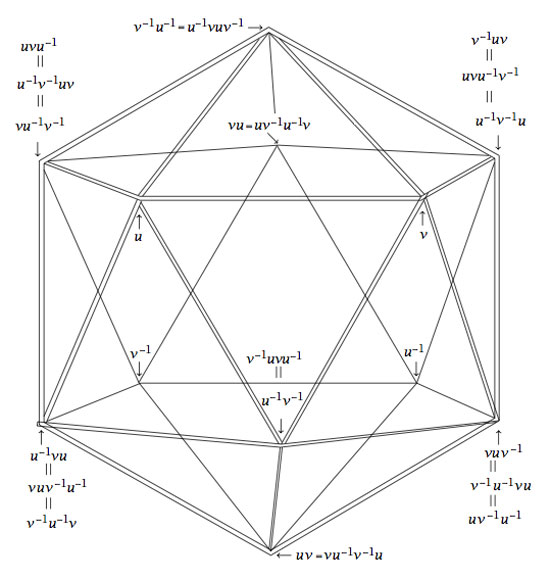Isn’t that nice? Well yes, you may say, but that is just the icosahedron. Can the group $A_5$ also see the buckyball?

Well, let’s try a similar strategy : the buckyball has 60 vertices, exactly as many as there are elements in the group $A_5$. Is there a way to connect certain elements in a group according to fixed rules? Yes, there is such a way and it is called the Cayley Graph of a group. It goes like this : take a set of generators ${ g_1,\ldots,g_k }$ of a group G, then connect two group element $a,b \in G$ with an edge if and only if $a = g_i.b$ or $b = g_i.a$ for some of the generators.

Back to the alternating group $A_5$. There are several sets of generators, one of them being the elements ${ (1,2,3,4,5),(2,3)(4,5) }$. In the paper mentioned before, Kostant gives an impressive group-theoretic proof of the fact that the Cayley-graph of $A_5$ with respect to these two generators is indeed the buckyball!

Let us allow to be lazy for once and let SAGE do the hard work for us, and let us just watch the outcome. Here’s how that’s done

A=PermutationGroup([‘(1,2,3,4,5)’,'(2,3)(4,5)’])
B=A.cayley_graph()
B.show3d()

The outcone is a nice 3-dimensional picture of the buckyball. Below you can see a still, and, if you click on it you will get a 3-dimensional model of it (first click the ‘here’ link in the new window and then you’d better control-click and set the zoom to 200% before you rotate it)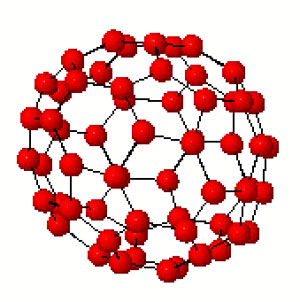Hence, viewing this Cayley graph from different points we have convinced ourselves that it is indeed the buckyball. In fact, most (truncated) Platonic solids appear as Cayley graphs of groups with respect to specific sets of generators. For later use here is a (partial) survey (taken from Jaap’s puzzle page)Tetrahedron : $C_2 \times C_2,[(12)(34),(13)(24),(14)(23)]$
Cube : $D_4,[(1234),(13)]$
Octahedron : $S_3,[(123),(12),(23)]$
Dodecahedron : IMPOSSIBLE
Icosahedron : $A_4,[(123),(234),(13)(24)]$Truncated tetrahedron : $A_4,[(123),(12)(34)]$
Cuboctahedron : $A_4,[(123),(234)]$
Truncated cube : $S_4,[(123),(34)]$
Truncated octahedron : $S_4,[(1234),(12)]$
Rhombicubotahedron : $S_4,[(1234),(123)]$
Rhombitruncated cuboctahedron : IMPOSSIBLE
Snub cuboctahedron : $S_4,[(1234),(123),(34)]$Icosidodecahedron : IMPOSSIBLE
Truncated dodecahedron : $A_5,[(124),(23)(45)]$
Truncated icosahedron : $A_5,[(12345),(23)(45)]$
Rhombicosidodecahedron : $A_5,[(12345),(124)]$
Rhombitruncated icosidodecahedron : IMPOSSIBLE
Snub Icosidodecahedron : $A_5,[(12345),(124),(23)(45)]$

Again, all these statements can be easily verified using SAGE via the method described before. Next time we will go further into the Kostant’s group-theoretic proof that the buckyball is the Cayley graph of $A_5$ with respect to (2,5)-generators as this calculation will be crucial in the description of the buckyball curve, the genus 70 Riemann surface discovered by David Singerman and
Pablo Martin which completes the trinity corresponding to the Galois trinityThere are only a handful of human activities where one goes to extraordinary lengths to keep a dream alive, in spite of overwhelming evidence : religion, theoretical physics, supporting the Belgian football team and … mathematics.

In recent years several people spend a lot of energy looking for properties of an elusive object : the field with one element $\mathbb{F}_1$, or in French : “F-un”. The topic must have reached a level of maturity as there was a conference dedicated entirely to it : NONCOMMUTATIVE GEOMETRY AND GEOMETRY OVER THE FIELD WITH ONE ELEMENT.

In this series I’d like to find out what the fuss is all about, why people would like it to exist and what it has to do with noncommutative geometry. However, before we start two remarks :

The field $\mathbb{F}_1$ does not exist, so don’t try to make sense of sentences such as “The ‘field with one element’ is the free algebraic monad generated by one constant (p.26), or the universal generalized ring with zero (p.33)” in the wikipedia-entry. The simplest proof is that in any (unitary) ring we have $0 \not= 1$ so any ring must contain at least two elements. A more highbrow version : the ring of integers $\mathbb{Z}$ is the initial object in the category of unitary rings, so it cannot be an algebra over anything else.

The second remark is that several people have already written blog-posts about $\mathbb{F}_1$. Here are a few I know of : David Corfield at the n-category cafe and at his old blog, Noah Snyder at the secret blogging seminar, Kea at the Arcadian functor, AC and K. Consani at Noncommutative geometry and John Baez wrote about it in his weekly finds.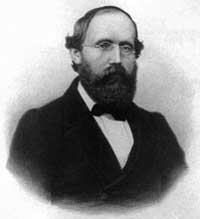The dream we like to keep alive is that we will prove the Riemann hypothesis one fine day by lifting Weil’s proof of it in the case of curves over finite fields to rings of integers.

Even if you don’t know a word about Weil’s method, if you think about it for a couple of minutes, there are two immediate formidable problems with this strategy.

For most people this would be evidence enough to discard the approach, but, we mathematicians have found extremely clever ways for going into denial.

The first problem is that if we want to think of $\mathbf{spec}(\mathbb{Z})$ (or rather its completion adding the infinite place) as a curve over some field, then $\mathbb{Z}$ must be an algebra over this field. However, no such field can exist…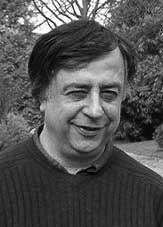No problem! If there is no such field, let us invent one, and call it $\mathbb{F}_1$. But, it is a bit hard to do geometry over an illusory field. Christophe Soule succeeded in defining varieties over $\mathbb{F}_1$ in a talk at the 1999 Arbeitstagung and in a more recent write-up of it : Les varietes sur le corps a un element.

We will come back to this in more detail later, but for now, here’s the main idea. Consider an existent field $k$ and an algebra $k \rightarrow R$ over it. Now study the properties of the functor (extension of scalars) from $k$-schemes to $R$-schemes. Even if there is no morphism $\mathbb{F}_1 \rightarrow \mathbb{Z}$, let us assume it exists and define $\mathbb{F}_1$-varieties by requiring that these guys should satisfy the properties found before for extension of scalars on schemes defined over a field by going to schemes over an algebra (in this case, $\mathbb{Z}$-schemes). Roughly speaking this defines $\mathbb{F}_1$-schemes as subsets of points of suitable $\mathbb{Z}$-schemes.

But, this is just one half of the story. He adds to such an $\mathbb{F}_1$-variety extra topological data ‘at infinity’, an idea he attributes to J.-B. Bost. This added feature is a $\mathbb{C}$-algebra $\mathcal{A}_X$, which does not necessarily have to be commutative. He only writes : “Par ignorance, nous resterons tres evasifs sur les proprietes requises sur cette $\mathbb{C}$-algebre.”The algebra $\mathcal{A}_X$ originates from trying to bypass the second major obstacle with the Weil-Riemann-strategy. On a smooth projective curve all points look similar as is clear for example by noting that the completions of all local rings are isomorphic to the formal power series $k[[x]]$ over the basefield, in particular there is no distinction between ‘finite’ points and those lying at ‘infinity’.

The completions of the local rings of points in $\mathbf{spec}(\mathbb{Z})$ on the other hand are completely different, for example, they have residue fields of different characteristics… Still, local class field theory asserts that their quotient fields have several common features. For example, their Brauer groups are all isomorphic to $\mathbb{Q}/\mathbb{Z}$. However, as $Br(\mathbb{R}) = \mathbb{Z}/2\mathbb{Z}$ and $Br(\mathbb{C}) = 0$, even then there would be a clear distinction between the finite primes and the place at infinity…

Alain Connes came up with an extremely elegant solution to bypass this problem in Noncommutative geometry and the Riemann zeta function. He proposes to replace finite dimensional central simple algebras in the definition of the Brauer group by AF (for Approximately Finite dimensional)-central simple algebras over $\mathbb{C}$. This is the origin and the importance of the Bost-Connes algebra.

We will come back to most of this in more detail later, but for the impatient, Connes has written a paper together with Caterina Consani and Matilde Marcolli Fun with $\mathbb{F}_1$ relating the Bost-Connes algebra to the field with one element.

I’ve always thought of Alain Connes as the unchallengeable world-champion opaque mathematical writing, but then again, I was proven wrong.

Alain’s writings are crystal clear compared to the monstrosity the AMS released to the world : In search of the Riemann zeros – Strings, fractal membranes and noncommutative spacetimes by Michel L. Lapidus.

Here’s a generic half-page from a total of 558 pages (or rather 314, as the remainder consists of appendices, bibliography and indices…). I couldn’t find a single precise, well-defined and proven statement in the entire book.

4.2. Fractal Membranes and the Second Quantization of Fractal Strings
“The first quantization is a mystery while the second quantization is a functor” Edward Nelson (quoted in [Con6,p.515])

We briefly discuss here joint work in preparation with Ryszard Nest [LapNe1]. This work was referred to several times in Chapter 3, and, as we pointed out there, it provides mathematically rigorous construction of fractal membranes (as well as of self-similar membranes), in the spirit of noncommutative geometry and quantum field theory (as well as of string theory). It also enables us to show that the expected properties of fractal (or self-similar) membranes, derived in our semi-heuristic model presented in Sections 3.2 and 3.2. are actually satisfied by the rigorous model in [LapNe1]. In particular, there is a surprisingly good agreement between the author’s original intuition on fractal (or self-similar) membrane, conceived as an (adelic) Riemann surface with infinite genus or as an (adelic) infinite dimensional torus, and properties of the noncommutative geometric model in [LapNe1]. In future joint work, we hope to go beyond [LapNe1] and to give even more (noncommutative) geometric content to this analogy, possibly along the lines suggested in the next section (4.3).
We will merely outline some aspects of the construction, without supplying any technical details, instead referring the interested reader to the forthcoming paper [LapNe1] for a complete exposition of the construction and precise statements of results.

Can the AMS please explain to the interested person buying this book why (s)he will have to await a (possible) forthcoming paper to (hopefully) make some sense of this apparent nonsense?

Time to wrap up this series on the Bost-Connes algebra. Here’s what we have learned so far : the convolution product on double cosets

$\begin{bmatrix} 1 & \mathbb{Z} \\ 0 & 1 \end{bmatrix} \backslash \begin{bmatrix} 1 & \mathbb{Q} \\ 0 & \mathbb{Q}_{> 0} \end{bmatrix} / \begin{bmatrix} 1 & \mathbb{Z} \\ 0 & 1 \end{bmatrix}$

is a noncommutative algebra, the Bost-Connes Hecke algebra $\mathcal{H}$, which is a bi-chrystalline graded algebra (somewhat weaker than ‘strongly graded’) with part of degree one the group-algebra $\mathbb{Q}[\mathbb{Q}/\mathbb{Z}]$. Further, $\mathcal{H}$ has a natural one-parameter family of algebra automorphisms $\sigma_t$ defined by $\sigma_t(X_n) = n^{it}X_n$ and $\sigma_t(Y_{\lambda})=Y_{\lambda}$.

For any algebra $A$ together with a one-parameter family of automorphisms $\sigma_t$ one is interested in KMS-states or Kubo-Martin-Schwinger states with parameter $\beta$, $KMS_{\beta}$ (this parameter is often called the ‘invers temperature’ of the system) as these are suitable equilibria states. Recall that a state is a special linear functional $\phi$ on $A$ (in particular it must have norm one) and it belongs to $KMS_{\beta}$ if the following commutation relation holds for all elements $a,b \in A$

$\phi(a \sigma_{i\beta}(b)) = \phi(b a)$

Let us work out the special case when $A$ is the matrix-algebra $M_n(\mathbb{C})$. To begin, all algebra-automorphisms are inner in this case, so any one-parameter family of automorphisms is of the form

$\sigma_t(a) = e^{itH} a e^{-itH}$

where $e^{itH}$ is the matrix-exponential of the nxn matrix $H$. For any parameter $\beta$ we claim that the linear functional

$\phi(a) = \frac{1}{tr(e^{-\beta H})} tr(a e^{-\beta H})$

is a KMS-state.Indeed, we have for all matrices $a,b \in M_n(\mathbb{C})$ that

$\phi(a \sigma_{i \beta}(b)) = \frac{1}{tr(e^{-\beta H})} tr(a e^{- \beta H} b e^{\beta H} e^{- \beta H})$

$= \frac{1}{tr(e^{-\beta H})} tr(a e^{-\beta H} b) = \frac{1}{tr(e^{-\beta H})} tr(ba e^{-\beta H}) = \phi(ba)$

(the next to last equality follows from cyclic-invariance of the trace map).
These states are usually called Gibbs states and the normalization factor $\frac{1}{tr(e^{-\beta H})}$ (needed because a state must have norm one) is called the partition function of the system. Gibbs states have arisen from the study of ideal gases and the place to read up on all of this are the first two chapters of the second volume of “Operator algebras and quantum statistical mechanics” by Ola Bratelli and Derek Robinson.

This gives us a method to construct KMS-states for an arbitrary algebra $A$ with one-parameter automorphisms $\sigma_t$ : take a simple n-dimensional representation $\pi~:~A \mapsto M_n(\mathbb{C})$, find the matrix $H$ determining the image of the automorphisms $\pi(\sigma_t)$ and take the Gibbs states as defined before.

Let us return now to the Bost-Connes algebra $\mathcal{H}$. We don’t know any finite dimensional simple representations of $\mathcal{H}$ but, sure enough, have plenty of graded simple representations. By the usual strongly-graded-yoga they should correspond to simple finite dimensional representations of the part of degree one $\mathbb{Q}[\mathbb{Q}/\mathbb{Z}]$ (all of them being one-dimensional and corresponding to characters of $\mathbb{Q}/\mathbb{Z}$).

Hence, for any $u \in \mathcal{G} = \prod_p \hat{\mathbb{Z}}_p^{\ast}$ (details) we have a graded simple $\mathcal{H}$-representation $S_u = \oplus_{n \in \mathbb{N}_+} \mathbb{C} e_n$ with action defined by

$\begin{cases} \pi_u(X_n)(e_m) = e_{nm} \\ \pi_u(Y_{\lambda})(e_m) = e^{2\pi i n u . \lambda} e_m \end{cases}$

Here, $u.\lambda$ is computed using the ‘chinese-remainder-identification’ $\mathcal{A}/\mathcal{R} = \mathbb{Q}/\mathbb{Z}$ (details).

Even when the representations $S_u$ are not finite dimensional, we can mimic the above strategy : we should find a linear operator $H$ determining the images of the automorphisms $\pi_u(\sigma_t)$. We claim that the operator is defined by $H(e_n) = log(n) e_n$ for all $n \in \mathbb{N}_+$. That is, we claim that for elements $a \in \mathcal{H}$ we have

$\pi_u(\sigma_t(a)) = e^{itH} \pi_u(a) e^{-itH}$

So let us compute the action of both sides on $e_m$ when $a=X_n$. The left hand side gives $\pi_u(n^{it}X_n)(e_m) = n^{it} e_{mn}$ whereas the right-hand side becomes

$e^{itH}\pi_u(X_n) e^{-itH}(e_m) = e^{itH} \pi_u(X_n) m^{-it} e_m =$

$e^{itH} m^{-it} e_{mn} = (mn)^{it} m^{-it} e_{mn} = n^{it} e_{mn}$

proving the claim. For any parameter $\beta$ this then gives us a KMS-state for the Bost-Connes algebra by

$\phi_u(a) = \frac{1}{Tr(e^{-\beta H})} Tr(\pi_u(a) e^{-\beta H})$

Finally, let us calculate the normalization factor (or partition function) $\frac{1}{Tr(e^{-\beta H})}$. Because $e^{-\beta H}(e_n) = n^{-\beta} e_n$ we have for that the trace

$Tr(e^{-\beta H}) = \sum_{n \in \mathbb{N}_+} \frac{1}{n^{\beta}} = \zeta(\beta)$

is equal to the Riemann zeta-value $\zeta(\beta)$ (at least when $\beta > 1$).

Summarizing, we started with the definition of the Bost-Connes algebra $\mathcal{H}$, found a canonical one-parameter subgroup of algebra automorphisms $\sigma_t$ and computed that the natural equilibria states with respect to this ‘time evolution’ have as their partition function the Riemann zeta-function. Voila!